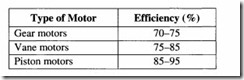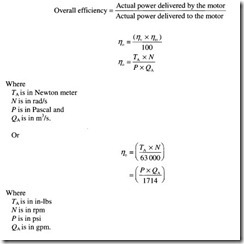# Hydraulic motors:Hydraulic motor performance

###### Hydraulic motor performance

The performance of a hydraulic motor depends on a lot of factors such as:

• Manufacturing precision

• Maintenance of close tolerances

• Internalleakage

• Friction between mating parts and

• Internal fluid turbulence.

While internal leakage between the motor inlet and outlet leads to decreased volumetric efficiency, loss in mechanical efficiency occurs on account of friction between the mating parts and also due to fluid turbulence.

The table below shows the typical overall efficiencies for gear, vane and piston motors.Hydraulic motor performance is evaluated on the basis of the same basic parameters of volumetric efficiency, mechanical efficiency and overall efficiency, similar to that of hydraulic pumps.

###### Volumetric efficiency

The volumetric efficiency (‘7v) of a hydraulic motor is given by

Where

QT is equal to the theoretical flow rate the motor should consume and

QA is the actual flow rate consumed by the motor.

From the above equation, the volumetric efficiency of a hydraulic motor is found to be the exact inverse of that of a hydraulic pump. The reason for this being that a motor consumes more flow than it should theoretically, on account of leakage, whereas a pump does not produce as much flow as it should theoretically.

The volumetric efficiency can be determined, if the values of QT and QA are known. While QT, the theoretical flow rate can be determined with the help of the equation derived earlier, QA, the actual flow rate is measured.

###### Mechanical efficiency

The mechanical efficiency (17m) of a hydraulic motor is given by

From the above equations, the mechanical efficiency of a hydraulic motor is again found to be the exact inverse of that of a hydraulic pump. This is because a pump requires greater torque than it should theoretically, on account of friction, whereas lesser torque is produced by the motor than it should theoretically.

Overall efficiency

The overall efficiency (1]0) for motors as in the case of pumps is given by the product of the volumetric and mechanical efficiencies.One important point to be noted is that while the actual power delivered to a motor by the fluid is known as hydraulic power, the actual power delivered by the motor to a load through a rotating shaft is known as brake power.